# The Golden Ratio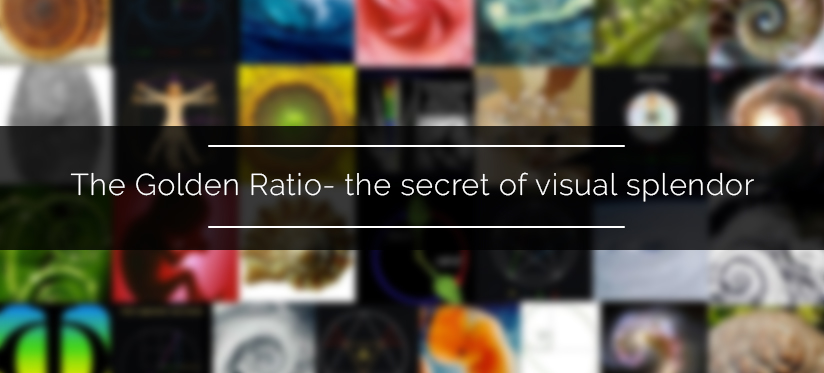Have you heard of the concept called – mathematically beautiful?

We rarely equate math with beauty and elegance. Though the concept of beauty is very relative, it can be related to the aspects of ratio, proportion and placement defining perfection. Scientists through ages have noted some astonishing facts such as the natural geometric structures or a series of sequence in the natural beings. Likewise, Golden Ratio has been heralded as the most beautiful ratio in art and architecture for centuries.

WHAT IS GOLDEN RATIO?

The Golden ratio is a special number found by dividing a line into two parts so that the longer part divided by the smaller part is also equal to the whole length divided by the longer part.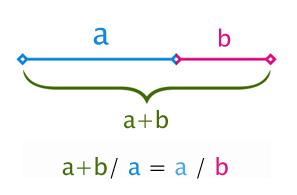It is often symbolized using phi, after the 21st letter of the Greek alphabet. In an equation form, it looks like this:

a/b = (a+b)/a = 1.6180339887498948420 …

As with Phi (the ratio of the circumference of a circle to its diameter), the digits go on and on, theoretically into infinity. Phi is usually rounded off to 1.618. This number has been discovered and rediscovered many times, which is why it has many other versions of names such as the Golden mean, the Golden section, divine proportion, etc.

SOME HISTORY NOW

One can find the application of Golden Ratio in the architecture of many ancient civilizations.

• Phidias, the Greek sculptor and mathematician is thought to have applied the golden ratio to the design of sculptures for the Parthenon.
• Plato stated Golden Ratio to be the most universally binding of mathematical relationships.
• Euclid had linked the Golden Ratio to the construction of a pentagram
• It has been used to achieve balance and beauty in many Renaissance paintings and sculptures
• Leonardo Da Vinci has incorporated the Golden Ratio to define all of the proportions in his Last Supper including the dimensions of the table and the proportions of the walls as well as the background.

Let’s take an example and observe how this magical ratio is been utilized and proved to be a proportion of beauty and elegance of an architecture.

PARTHENON

Parthenon, the famous temple in Athens, is an oblivious dust of the beautiful architecture from the ancient Europe. Built by ancient Greeks from 447 to 438 BC, it is regarded by many to illustrate the application of the Golden Ratio in its design.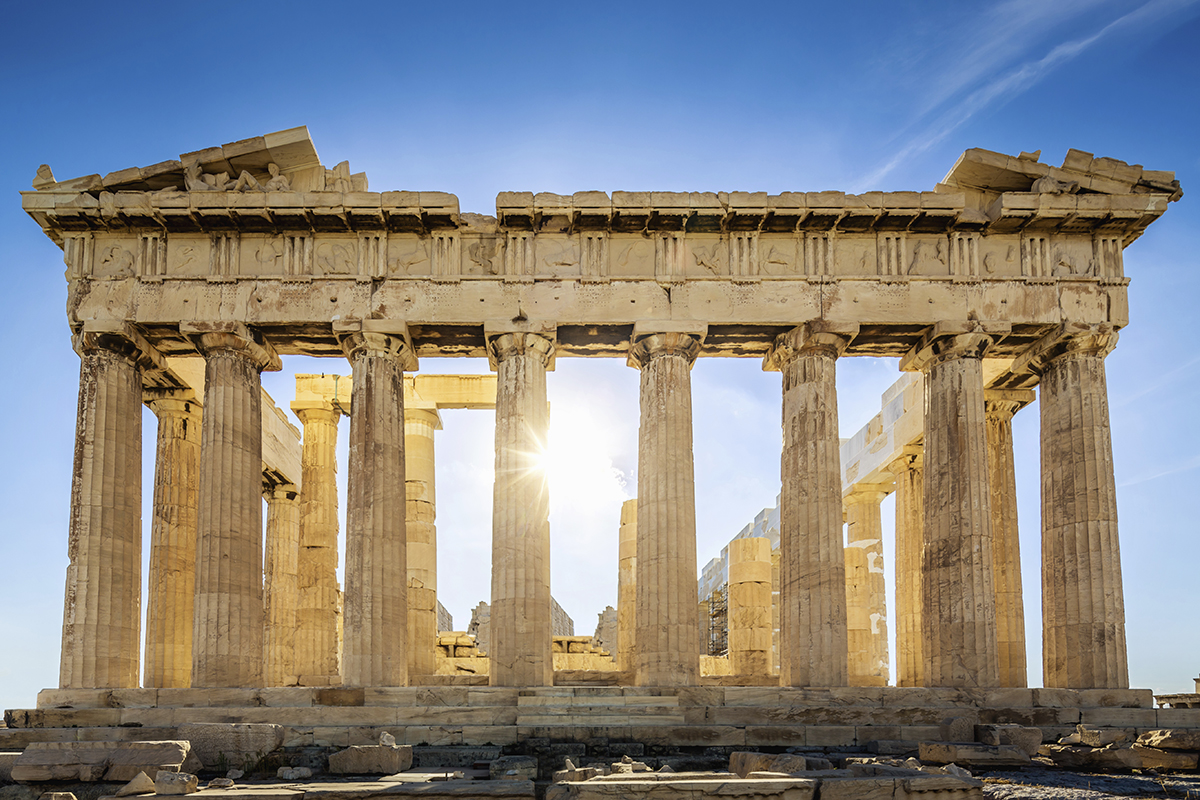The picture above shows the front face of the Parthenon, standing tall as the evidence of the great history. It was finished in 432 B.C and was a temple which was destroyed by the Persians in 480 B.C. The thousand years old temple was then turned into a church by the Roman Emperors. It has witnessed many major events in the history and survived its way to this modern era. The above-shown picture is the surviving bit which was then partially restored by the Greek government back in 1975.

The photo below shows a Golden Rectangles with a Golden Spiral overlay to the entire face of the Parthenon.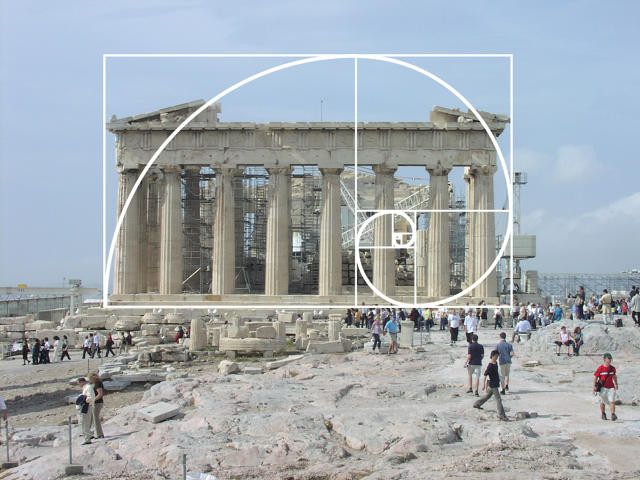This illustrates that the height and width of the Parthenon conform closely to Golden Ratio proportions. This construction requires an assumption though, that the bottom of the golden rectangle should align with the bottom of the second step into the structure and that the top should align with a peak of the roof that is projected by the remaining sections.  Given that assumption, the top of the columns and base of the roof line are in a close golden ratio proportion to the height of the Parthenon.  The ratio of length to the height of the entire rectangle is l : h. When we divide the length into two parts as mentioned, we get a square of side h, and a rectangle of dimensions h by l-h. The ratio of the height of this rectangle to its length will now be h : l-h. Now, this ratio is exactly equal to the ratio l : h, the ratio of the sides of the original rectangle. Intriguing, isn’t it?

The interesting fact is that it is not just the entire front face of Parthenon which was built in the Golden Ratio, but also the spacing between consecutive columns. Every minute details of the structure had incorporated this ratio.

Two numbers are said to be in the golden ratio if the ratio of their sum to the greater number is equal to the ratio of the larger number to the smaller one. So if a and b are in the golden ratio, then.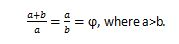Now the value of ϕ is calculated to be equal to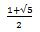,which is roughly 1.618. The calculation is simple, as, from the previous equation, we obtain a quadratic equation ϕ2-ϕ-1 = 0, from which we get the mentioned value.

FIBONACCI SEQUENCE AND NATURE

The golden ratio is closely associated with the Fibonacci sequence too. Around 1200, the mathematician Leonardo Fibonacci discovered the unique properties of this sequence.

The sequence starts from 0, 1, 1, 2, 3, 5, 8, 13, 21 and so on.

This magical sequence ties directly with the Golden Ratio because if you take any two successive Fibonacci numbers, their ratio is very close to the Golden Ratio. As the numbers get higher, the ratio becomes even closer to 1.625.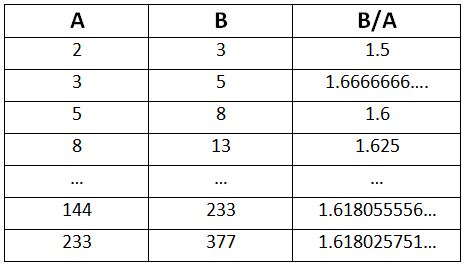The Golden ratio also appears in all forms of nature and science. Some unexpected places include Flower petals, seed heads, Tree branches, shells, spiral galaxies etc.

Take a look at the pattern of petals of any flower. The number of the petals in a flower consistently follows the Fibonacci sequence. Some common examples include the lily, which had three petals, buttercups which have five petals.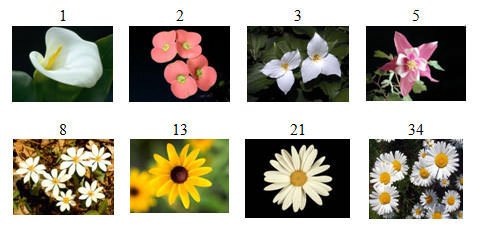So, the fundamental answer to the indescribable beauty of any structure or a collection of forms is the Golden ratio. Anything elegant and attractive on this earth has some strings attached to this magical ratio. Now we know a fact that beauty could be brought using Mathematics.

Sudhanshu kondekar

December 1, 2017

I’ve read this in THE DA VINCI CODE BY THE GREAT AUTHOR
Dan Brown

Testimonials

### Book Your Free Class Now